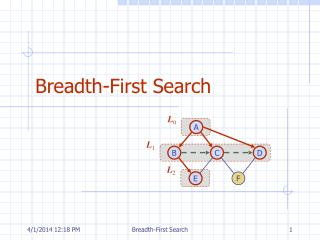DownloadDownload PresentationTélécharger la présentation- - - - - - - - - - - - - - - - - - - - - - - - - - - E N D - - - - - - - - - - - - - - - - - - - - - - - - - - -
##### Presentation Transcript

1. L0 A L1 B C D L2 E F Breadth-First Search Breadth-First Search

2. Outline and Reading • Breadth-first search (§6.3.3) • Algorithm • Example • Properties • Analysis • Applications • DFS vs. BFS (§6.3.3) • Comparison of applications • Comparison of edge labels Breadth-First Search

3. Breadth-first search (BFS) is a general technique for traversing a graph A BFS traversal of a graph G Visits all the vertices and edges of G Determines whether G is connected Computes the connected components of G Computes a spanning forest of G BFS on a graph with n vertices and m edges takes O(n + m ) time BFS can be further extended to solve other graph problems Find and report a path with the minimum number of edges between two given vertices Find a simple cycle, if there is one Breadth-First Search Breadth-First Search

4. L0 A L1 B C D E F Example unexplored vertex A visited vertex A unexplored edge discovery edge cross edge L0 L0 A A L1 L1 B C D B C D E F E F Breadth-First Search

5. L0 L0 A A L1 L1 B C D B C D L2 E F E F L0 L0 A A L1 L1 B C D B C D L2 L2 E F E F Example (cont.) Breadth-First Search

6. L0 L0 A A L1 L1 B C D B C D L2 L2 E F E F Example (cont.) L0 A L1 B C D L2 E F Breadth-First Search

7. BFS Algorithm AlgorithmBFS(G, s) L0new empty sequence L0.insertLast(s) setLabel(s, VISITED) i  0 while Li.isEmpty() Li +1new empty sequence for all v  Li.elements() for all e  G.incidentEdges(v) ifgetLabel(e) = UNEXPLORED w opposite(v,e) if getLabel(w) = UNEXPLORED setLabel(e, DISCOVERY) setLabel(w, VISITED) Li +1.insertLast(w) else setLabel(e, CROSS) i  i +1 • The algorithm uses a mechanism for setting and getting “labels” of vertices and edges AlgorithmBFS_all(G) Inputgraph G Outputlabeling of the edges and partition of the vertices of G for all u  G.vertices() setLabel(u, UNEXPLORED) for all e  G.edges() setLabel(e, UNEXPLORED) for all v  G.vertices() ifgetLabel(v) = UNEXPLORED BFS(G, v) Breadth-First Search

8. Notation Gs: connected component of s Property 1 BFS(G, s) visits all the vertices and edges of Gs Property 2 The discovery edges labeled by BFS(G, s) form a spanning tree Ts of Gs Property 3 For each vertex v in Li The path of Ts from s to v has i edges Every path from s to v in Gshas at least i edges Properties A B C D E F L0 A L1 B C D L2 E F Breadth-First Search

9. Setting/getting a vertex/edge label takes O(1) time Each vertex is labeled twice once as UNEXPLORED once as VISITED Each edge is labeled twice once as UNEXPLORED once as DISCOVERY or CROSS Each vertex is inserted once into a sequence Li Method incidentEdges is called once for each vertex BFS runs in O(n + m) time provided the graph is represented by the adjacency list structure Recall that Sv deg(v)= 2m Analysis Breadth-First Search

10. Applications • Using the template method pattern, we can specialize the BFS traversal of a graph Gto solve the following problems in O(n + m) time • Compute the connected components of G • Compute a spanning forest of G • Find a simple cycle in G, or report that G is a forest • Given two vertices of G, find a path in G between them with the minimum number of edges, or report that no such path exists Breadth-First Search

11. L0 A L1 B C D L2 E F DFS vs. BFS A B C D E F DFS BFS Breadth-First Search

12. Back edge(v,w) w is an ancestor of v in the tree of discovery edges Cross edge(v,w) w is in the same level as v or in the next level in the tree of discovery edges L0 A L1 B C D L2 E F DFS vs. BFS (cont.) A B C D E F DFS BFS Breadth-First Search